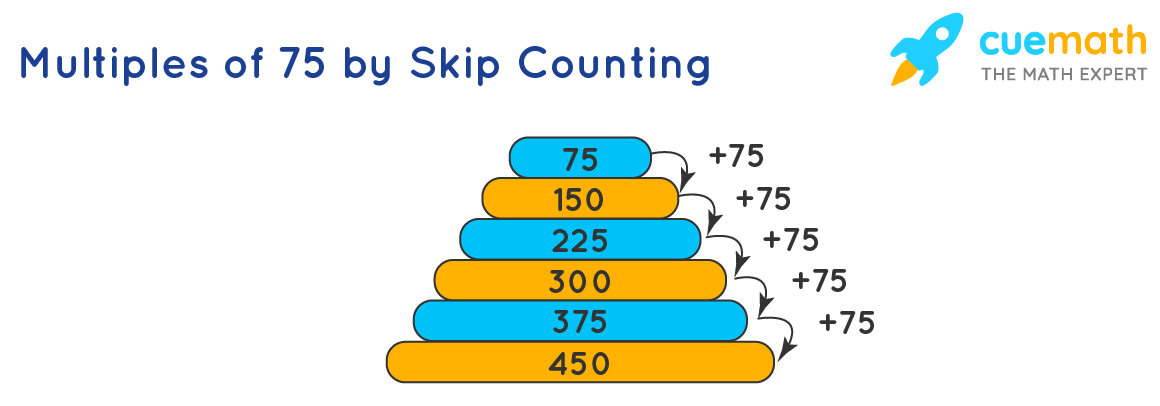# Multiples of 75

Did you know the number 75 is an odd composite number which is obtained from product of two prime numbers? In this mini-lesson, we will learn about the multiples of 75, and interesting facts about these multiples with solved examples and interactive questions.

• First five multiples of 75: 75, 150, 225, 300, 375
• Prime Factorization of 75: 75 = 3 × 5 × 5 = 3 × 52

## What are the Multiples of 75?

The multiples of 75 are numbers that are obtained by multiplying 75 by any integer.The first five multiples of 75 are 75, 150, 225, 300, and 375.

## List of First 20 Multiples of 75

The first 20 multiples of the number 75 are listed in the table below.

Multiply 75 by the numbers from 1 to 20 Multiples of 75
75 × 1 75
75 × 2 150
75 × 3 225
75 × 4 300
75 × 5 375
75 × 6 450
75 × 7 525
75 × 8 600
75 × 9 675
75 × 10 750
75 × 11 825
75 × 12 900
75 × 13 975
75 × 14 1050
75 × 15 1125
75 × 16 1200
75 × 17 1275
75 × 18 1350
75 × 19 1425
75 × 20 1500

To understand the concept of finding multiples, let us take a few more examples.

• Multiples of 12 - The first five multiples of 12 are 12, 24, 36, 48, 60
• Multiples of 25 - The first five multiples of 25 are 25, 50, 75, 100, 125
• Multiples of 30 - The first five multiples of 30 are 30, 60, 90, 120, 150
• Multiples of 8 - The first five multiples of 8 are 8, 16, 24, 32, 40
• Multiples of 7 - The first five multiples of 7 are 7, 14, 21, 28, 35

## FAQs on Multiples of 75

### How many multiples does 75 Have?

The number of multiples of any number is infinite. eg: 75, 150, 225, 300, 375 etc.

### Are the multiples of 75 always odd?

No, the multiples of 75 are not always odd. For example 150, 300, are even numbers and are the multiples of 75 as well.

### Is 3 a Multiple of 75?

No, 3 is not a multiple of 75.

### What is the Least Common Multiple of 75 and 12?

The least common multiples of 75 and 12 can be calculated by: (75 × 12)/GCF(75, 12). Here GCF(a,b) is the greatest common factors of 75 and 12.
GCF(75, 12) = 3
LCM(75, 12) = (75 × 12)/GCF(75, 12) = 75 × 4 = 300

### What is the Least Common Multiple of 75 and 180 ?

The least common multiples of two numbers 75 and 180 can be calculated using the formula: (75 × 180)/GCF(75,180).
GCF(75, 180) = 15
LCM(75, 180) = (75 × 180)/GCF(75, 180) = 5 × 180 = 900

Challenging Questions:

• Is 75 a multiple of:
a) 7.5
b) 22.5?
• Find the even multiples of 175?

## Multiples of 75 Solved Examples

Example 1:  Sean has about 600 chocolates, which have to be filled in boxes containing 75 each.  How many boxes would Sean need to pack all the chocolates?

Solution:

Total number of chocolates present with Sean is 600. Capacity of each box is 75 per box.
Number of boxes needed to pack all the chocolates = 600/75 = 8.
Hence, Sean would need 8 boxes.

Example 2:  During the weekend, Jacky plans to drive to meet his parents. He estimates that it would take about 75 hours to drive this distance. Calculate the travel time of Jacky in minutes.

Solution:

Time taken by Jacky to drive = 75 hours
Number of minutes in 1 hour = 60 minutes
Time taken to travel (in minutes) = 75 × 60  = 4500 minutes.
Hence, Jacky would take a total of 4500 minutes.

Example 3:  Jonathan is a builder, and he wishes to fence his land. Also, he wants to have every fifth pole of the fence in blue color. If Jonathan has used 75 blue poles for fencing his land, find the total number of poles used in the fence.

Solution:

Jonathan wishes to have every fifth pole of the fence in blue color. A set of 5 poles would have 4 poles without blue color and the 5th pole in blue color.
Number of blue poles used in the fence is 75. Total number of poles = 75 × 5 = 375

## Interactive Questions

Here are a few activities for you to practice. Select/Type your answer and click the "Check Answer" button to see the result.

Think Tank:

• Among the multiples of 75, what position is 1500 at?
• Is the product of two or more factors the multiple of each factor?

More Important Topics
Numbers
Algebra
Geometry
Measurement
Money
Data
Trigonometry
Calculus